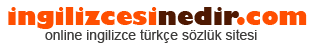ingilizce türkçe sözlük sitesine hoşgeldiniz.| Ana Sayfaİngilizce Türkçe Sözlükte Ara: Türkçe->İngilizce İngilizce->Türkçe * Aramak istediğiniz kelimeyi yazıp dil seçimini seçtikten sonra kelimenin anlamını öğrenebilirsiniz.

Kelime Anlamı

## Türkçeden İngilizceye Çeviri Sonucu

• Unit of electrical capacity; capacity of a condenser which, when charged with one coulomb of electricity, gives difference of potential of one volt. the capacitance of a capacitor that has an equal and opposite charge of 1 coulomb on each plate and a voltage difference of 1 volt between the plates.
• The basic unit of capacitance A capacitor has a value of one farad when it can store one coulomb of charge with one volt across it.
• The standard unit of capacitance Abbreviated as F A voltage change of one volt per second produces a current of one amp across a one-farad capacitance The farad is too large a unit for practical application, so two smaller units are generally used The microfarad is equal to 10-6 F, and the picofarad is 10- 2 F.
• The SI unit of capacitance A farad is the capacitance of a capacitor in which a charge of 1 coulomb produces a potential difference of 1 volt between the terminals.
• A unit of electrical capacity.
• The unit of capacitance.
• The unit of capacitance 'F' This is a very large value and the most common units are microfarad and nanofarad.
• The unit of capacity of a capacitor Capacitors in our industry are rated in microfarads. the basic unit of capacitance A capacitor has a capacitance of 1 farad when a voltage change of 1 volt per second across it produces a current of 1 ampere. the SI unit of capacitance, named after Michael Faraday, symbol F One farad is a very large capacitance; values of capacitors used in typical circuits are in the microfarad range.
• The unit of electrical capacitance; the energy storing capacity of a capacitor. unit of measure of capacitance Named after Farad.
• The basic unit of capacitance A capacitor has a capacitance of 1F when a charge of 1 volt across the capacitor produces a current of 1 ampere through it Named after Michael Faraday.
• A farad is the measure of capacitance One farad is equal to 1 coulomb of charge between two terminals causing one volt of potential difference.
• Unit of capacitance whereby a charge of one coulomb produces a one volt potential difference.
• The basic unit of capacitance A capacitor has a capacitance of 1F when a charge of 1 Volt across the capacitor produces a current of 1 Ampere through it Named after Michael Faraday 2.
• The unit for capacitance A capacitor that stored one coulomb of charge with one volt across it will have a value of one farad. the basic unit of capacitance A capacitor has a value of one farad when it can store one coulomb of charge with one volt across it.
• The capacitance of a capacitor in which a charge of 1 coulomb produces a change of 1 volt in the potential difference between its terminals The Farad is the unit of capacitance in the mksa system.
• The unit of capacitance; one coulomb per volt.
• A unit of capacity that will store one coulomb of electrical charge when one volt of electrical pressure is applied.
• The unit of electrical capacitance, the capacitance of a condenser between the plates of which there is a difference of potential of 1 volt when it is charged by a quantity of electricity equal to 1 coulomb.
• A unit of capacitance that stores one coulomb of electrical charge when one volt of electrical pressure is applied.
• The standard unit of electrical capacity; the capacity of a condenser whose charge, having an electro-motive force of one volt, is equal to the amount of electricity which, with the same electromotive force, passes through one ohm in one second; the capacity, which, charged with one coulomb, gives an electro-motive force of one volt. the capacitance of a capacitor that has an equal and opposite charge of 1 coulomb on each plate and a voltage difference of 1 volt between the plates.
Rastgele Türkçe Kelimeler